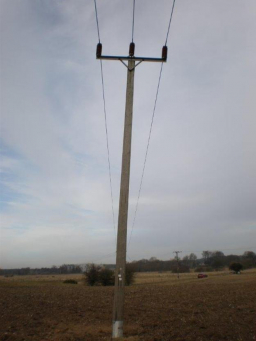# The lengths

The lengths of the twelve poles form an Arithmetic Progression (A. P). If the third pole is 3m and the eighth pole is 5 m, find the
(i) Length of the first pole
(ii) Sum of the length of the poles

a1 =  2.2 m
s =  52.8 m

### Step-by-step explanation:Did you find an error or inaccuracy? Feel free to write us. Thank you!

Tips for related online calculators
Need help calculating sum, simplifying, or multiplying fractions? Try our fraction calculator.
Do you have a linear equation or system of equations and looking for its solution? Or do you have a quadratic equation?
Do you want to convert length units?